# Complexification of a Lie group

(diff) ← Older revision | Latest revision (diff) | Newer revision → (diff)overThe complex Lie group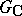containingas a real Lie subgroup such that the Lie algebraofis a real form of the Lie algebra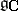of(see Complexification of a Lie algebra). One then says that the groupis a real form of the Lie group. For example, the group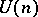of all unitary matrices of orderis a real form of the group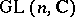of all non-singular matrices of orderwith complex entries.

There is a one-to-one correspondence between the complex-analytic linear representations of a connected simply-connected complex Lie groupand the real-analytic representations of its connected real form, under which irreducible representations correspond to each other. This correspondence is set up in the following way: Ifis an (irreducible) finite-dimensional complex-analytic representation of, then the restriction oftois an (irreducible) real-analytic representation of.

Not every real Lie group has a complexification. In particular, a connected semi-simple Lie group has a complexification if and only ifis linear, that is, is isomorphic to a subgroup of some group. For example, the universal covering of the group of real second-order matrices with determinant 1 does not have a complexification. On the other hand, every compact Lie group has a complexification.

The non-existence of complexifications for certain real Lie groups inspired the introduction of the more general notion of a universal complexification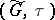of a real Lie group. Here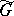is a complex Lie group and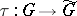is a real-analytic homomorphism such that for every complex Lie groupand every real-analytic homomorphism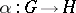there exists a unique complex-analytic homomorphismsuch that. The universal complexification of a Lie group always exists and is uniquely defined . Uniqueness means that if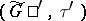is another universal complexification of, then there is a natural isomorphismsuch that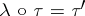. In general,, but ifis simply connected, then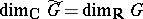and the kernel ofis discrete.